Updating search results...

# 195 Results

View
Selected filters:
• Math 2Rating
0.0 stars
Overview:

Sample Item provided by Smarter Balanced as preliminary examples of the types of items that students might encounter on the summative assessment. The item assesses whether students can perform arithmetic operations on polynomials. MAT.HS.ER.3.0AAPR.F.045

Subject:
Math 1
Math 2
Material Type:
Activity/Lab
Assessment
Provider:
Oregon Department of Education
Author:
Smarter Balanced Assessment Consortium
02/26/2019Rating
0.0 stars
Overview:

A Constructed Response Item provided by Smarter Balanced as preliminary examples of the types of items that students might encounter on the summative assessment. The item prompt students to produce a text or numerical response in order to collect evidence about their knowledge or understanding of representing constraints by equations and systems of equations, solving systems of linear equations and interpreting solutions as viable or nonviable options in a modeling context. MAT.HS.CR.2.0AREI.A.032

Subject:
Math 1
Math 2
Math 3
Material Type:
Activity/Lab
Assessment
Provider:
Oregon Department of Education
Author:
Smarter Balanced Assessment Consortium
02/26/2019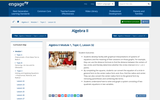Conditional Remix & Share Permitted
CC BY-NC-SA
Rating
0.0 stars
Overview:

Students develop facility with graphical interpretations of systems of equations and the meaning of their solutions on those graphs. For example, they can use the distance formula to find the distance between the centers of two circles and thereby determine whether the circles intersect in 0, 1, or 2 points.
By completing the squares, students can convert the equation of a circle in general form to the center-radius form and, thus, find the radius and center. They can also convert the center-radius form to the general form by removing parentheses and combining like terms.
Students understand how to solve and graph a system consisting of two quadratic equations in two variables.

Subject:
Math 1
Math 2
Material Type:
Lesson
Author:
EngageNY
02/23/2020Conditional Remix & Share Permitted
CC BY-NC-SA
Rating
0.0 stars
Overview:

Students understand the possibility that an equation—or a system of equations—has no real solutions. Students identify these situations and make the appropriate geometric connections.

Subject:
Math 2
Material Type:
Lesson
Author:
EngageNY
02/23/2020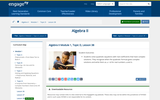Conditional Remix & Share Permitted
CC BY-NC-SA
Rating
0.0 stars
Overview:

Students solve quadratic equations with real coefficients that have complex solutions. They recognize when the quadratic formula gives complex solutions and write them as a + bi for real numbers a and b.

Subject:
Math 2
Material Type:
Lesson
Author:
EngageNY
02/23/2020Conditional Remix & Share Permitted
CC BY-NC-SA
Rating
0.0 stars
Overview:

Students solve quadratic equations with real coefficients that have complex solutions. Students extend polynomial identities to the complex numbers.
Students note the difference between solutions to the equation and the x-intercepts of the graph of said equation.

Subject:
Math 2
Material Type:
Lesson
Author:
EngageNY
02/23/2020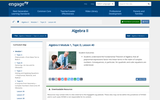Conditional Remix & Share Permitted
CC BY-NC-SA
Rating
0.0 stars
Overview:

Students understand the Fundamental Theorem of Algebra; that all polynomial expressions factor into linear terms in the realm of complex numbers. Consequences, in particular, for quadratic and cubic equations are understood.

Subject:
Math 2
Math 3
Material Type:
Lesson
Author:
EngageNY
02/23/2020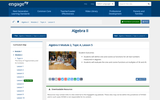Conditional Remix & Share Permitted
CC BY-NC-SA
Rating
0.0 stars
Overview:

Students will define sine and cosine as functions for all real numbers measured in degrees.
Students will evaluate the sine and cosine functions at multiples of 30 and 45.

Subject:
Math 2
Material Type:
Lesson
Author:
EngageNY
02/23/2020Rating
3.5 stars
Overview:

Using tiles to represent variables and constants, learn how to represent and solve algebra problem. Solve equations, substitute in variable expressions, and expand and factor. Flip tiles, remove zero pairs, copy and arrange, and make your way toward a better understanding of algebra.

Subject:
Mathematics
Math 1
Math 2
Math 3
Material Type:
Interactive
Provider:
National Council of Teachers of Mathematics
Author:
Illuminations
02/26/2019Conditional Remix & Share Permitted
CC BY-NC-SA
Rating
0.0 stars
Overview:

This is a ten question assessment afer a lesson taught on angle relationships and parallel lines.

Subject:
Math 2
Material Type:
Assessment
Author:
KORI ELLIOTT
07/14/2020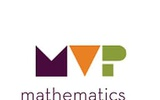Conditional Remix & Share Permitted
CC BY-NC-SA
Rating
0.0 stars
Overview:

The purpose of this task is to allow students to use their prior understandings of similar triangles to develop an understanding of trigonometric ratios. As the discussion about the trigonometric ratios and their usefulness surfaces, be sure to name the ratios with their names (sine, cosine, tangent) and demonstrate how they are most frequently written as cos, sin, tan.

Subject:
Math 2
Material Type:
Lesson
Author:
The Mathematics Vision Project
03/12/2020Conditional Remix & Share Permitted
CC BY-NC-SA
Rating
0.0 stars
Overview:

The purpose of this task is for students to connect the completing the square procedure learned in the previous two tasks to graphing parabolas. The task asks students to complete the square to change the equation of a quadratic function in standard form into vertex form. Students will need to extend their understanding of completing the square in an expression to an equation to maintain the equality, requiring that they add and subtract an equivalent term to one side of the equation (effectively adding zero) or that they add the same thing to both sides of the equation. After getting the equation into vertex form, students graph the equation of the parabola.

Subject:
Math 2
Material Type:
Lesson
Author:
The Mathematics Vision Project
03/11/2020Conditional Remix & Share Permitted
CC BY-NC-SA
Rating
0.0 stars
Overview:

The purpose of this task is for students to solidify their understanding about inverse functions. Students will understand how to find the inverse of a function and know when to restrict the domain so that they can produce an invertible function from a non-invertible function. Students will also become familiar with square root functions as a result of this task and will connect square root functions to their domain, range, and graphs.

Subject:
Math 2
Material Type:
Lesson
Author:
The Mathematics Vision Project
03/11/2020Rating
3.25 stars
Overview:

Susie is organizing the printing of tickets for a show. She has collected prices from several printers. Your task is to use a table and algebra to advise Susie on how to choose the best printer.

Subject:
Math 1
Math 2
Math 3
Material Type:
Activity/Lab
Assessment
Lesson Plan
Provider:
MARS, Mathematics Assessment Resource Service
Author:
Shell Center Team
02/26/2019Conditional Remix & Share Permitted
CC BY-NC-SA
Rating
0.0 stars
Overview:

The purpose of this task is for students to solidify their understanding of piecewise functions using their background knowledge of domain and linear functions. Students will solidify their understanding of piecewise functions by

• answering questions relating to a piecewise function
• using their knowledge of domain to graph each section of the piecewise function
• graphing complete piecewise-defined functions from equations
• interpreting the context of a piecewise function

Subject:
Math 2
Material Type:
Lesson
Author:
The Mathematics Vision Project
03/11/2020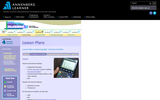Rating
5.0 stars
Overview:

Students explore quadratic functions by using a motion detector known as a Calculator Based Ranger (CBR) to examine the heights of the different bounces of a ball. Students will represent each bounce with a quadratic function of the form y = a (x - h)^2 + k. This lesson plan is based on the activity Tremain Nelson uses in the video for Part II of this workshop.

Subject:
Math 1
Math 2
Math 3
Material Type:
Lesson Plan
Provider:
Annenberg Learner
Author:
Annenberg Learner
02/26/2019Rating
0.0 stars
Overview:

In this lesson, students explore relationships between x-intercepts, factors, and roots of polynomial functions using the graphing calculator. Students also investigate rational functions, identifying the roots and the asymptotes as well as holes in the graphs. Students construct boxes of various dimensions using graph paper, collect height and volume data, and create a scatterplot in order to determine the height of the box with the maximum volume. Students can solve this problem using a graphing calculator or by using their own scatterplots drawn by hand. The use of questioning by the teacher, and the group work of the students are important features of this lesson.

Subject:
Math 1
Math 2
Math 3
Material Type:
Activity/Lab
Lesson Plan
Provider:
PBS
Author:
PBS Teachers Mathline
02/26/2019Conditional Remix & Share Permitted
CC BY-NC-SA
Rating
0.0 stars
Overview:

The purpose of this task is to develop students’ understanding of the procedure of completing the square using area models. In the task, students will use diagrams of area models to make sense of the terms in a perfect square trinomial and discover the relationship between coefficients of a quadratic expression. They will use this understanding to complete the square to find equivalent forms of quadratic expressions. In this task, the quadratic expressions will be limited to those in which the coefficient of the !! term is 1. This task is the beginning of a learning cycle that ends in students using the completing the square procedure to find the vertex form of a quadratic function and graph the associated parabola.

Subject:
Math 2
Material Type:
Lesson
Author:
The Mathematics Vision Project
03/11/2020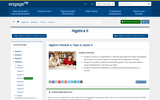Educational Use
Rating
3.0 stars
Overview:

Students learn a more formal definition of conditional probability and are asked to interpret conditional probabilities. Data are presented in two-way frequency tables, and conditional probabilities are calculated using column or row summaries.

Subject:
Math 2
Material Type:
Interactive
Provider:
EngageNY
Author:
EngageNY
04/04/2014Conditional Remix & Share Permitted
CC BY-NC-SA
Rating
0.0 stars
Overview:

In Math 8 students came to understand that a two-dimensional figure is congruent to another if the second can be obtained from the first by a sequence of rotations, reflections, and translations. In this task students are given two congruent figures and asked to describe a sequence of transformations that exhibits the congruence between them. While exploring potential sequences of transformations, students will notice how corresponding parts of congruent figures have to be carried onto one another, and they may look for ways that this can be accomplished in a consistent sequence of steps.

Subject:
Math 2
Material Type:
Lesson
Author:
The Mathematics Vision Project
03/10/2020Conditional Remix & Share Permitted
CC BY-NC-SA
Rating
0.0 stars
Overview:

In this task students are asked to develop a strategy for locating the center of a rotation, which leads to the observation that the center of rotation lies on the perpendicular bisectors of the segments joining image/pre-image pair of points. Such segments are defined to be chords of the circles on which the image/pre-image points are located. This work provides a method for finding the center of a circle. Students should also be able to prove that the perpendicular bisector of a chord contains a diameter of the circle and that the central angle formed by the radii that pass through the endpoints of the chord is bisected by the perpendicular diameter.

Subject:
Math 2
Material Type:
Lesson
Author:
The Mathematics Vision Project
03/12/2020Conditional Remix & Share Permitted
CC BY-NC-SA
Rating
0.0 stars
Overview:

The purpose of this task is to practice thinking through and contributing to the reasoning of others when working through a written geometric proof. Students will also practice all of the proof techniques discussed in this module: reasoning from a diagram; organizing a logical chain of reasoning from the given statements to the conclusion of the argument; and drawing upon postulates, definitions and previously proved statements to support the chain of reasoning. Three theorems are proved in this task:
• The medians of a triangle meet at a point.
• The angle bisectors of a triangle meet at a point.
• The perpendicular bisectors of the sides of a triangle meet at a point.

It is not necessary for students to create these theorems from scratch, but they should be able to follow and extend the reasoning of the arguments presented, based on the diagrams and previously proved theorems. The ideas proved here are novel and somewhat surprising. These theorems should demonstrate the power of deductive reasoning to establish the validity of ideas that at first seem counterintuitive.

Subject:
Math 2
Material Type:
Lesson
Author:
The Mathematics Vision Project
03/12/2020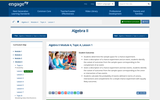Educational Use
Rating
0.0 stars
Overview:

Students determine the sample space for a chance experiment. Given a description of a chance experiment and an event, students identify the subset of outcomes from the sample space corresponding to the complement of an event. Given a description of a chance experiment and two events, students identify the subset of outcomes from the sample space corresponding to the union or intersection of two events. Students calculate the probability of events defined in terms of unions, intersections, and complements for a simple chance experiment with equally likely outcomes.

Subject:
Math 2
Material Type:
Interactive
Provider:
EngageNY
Author:
EngageNY
04/04/2014Conditional Remix & Share Permitted
CC BY-NC-SA
Rating
0.0 stars
Overview:

The purpose of this task is for students to interpret information provided that allows them to make sense of and organize data in a tree diagram, a two-way table, and a Venn diagram. Students will solidify their understanding of conditional probability by writing statements supported by data collected to justify the flavor of ice cream preferred by most. In this task, students will:
● Organize data into a tree diagram, two-way table, and a Venn diagram
● Calculate probabilities and conditional probabilities of A given B as the fraction of B’s outcomes that also belong to A, and interpret the answer in terms of the model ● Highlight the different representations and become more familiar with what each representation highlights and conceals.
● Continue to become more familiar with probability notation.
● Make decisions about meaning of data.

Subject:
Math 2
Material Type:
Lesson
Author:
The Mathematics Vision Project
03/12/2020Conditional Remix & Share Permitted
CC BY-NC-SA
Rating
0.0 stars
Overview:

The purpose of this and the following task is to give students practice in analyzing diagrams to identify conjectures, and then writing proofs to show that the conjectures are true. The specific theorems being examined in this and the following task are:
• Vertical angles are congruent • The measure of an exterior angle of a triangle is the sum of the two remote interior angles
• When a transversal crosses parallel lines, alternate interior angles are congruent, corresponding angles are congruent, and same-side interior angles are supplementary (add to 180°).

Subject:
Math 2
Material Type:
Lesson
Author:
The Mathematics Vision Project
03/12/2020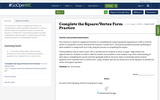Conditional Remix & Share Permitted
CC BY-NC
Rating
0.0 stars
Overview:

This resource is meant to supplement lessons on completing the square (quadratic equations) in order to find the vertex of a quadratic function and write the function in vertex form. The practice problems provide scaffolding to allow students to slowly build up to fully using the process of completing the square.

Subject:
Math 2
Material Type:
Activity/Lab
Author:
Nicole Smith
11/06/2019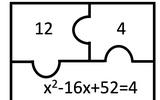Conditional Remix & Share Permitted
CC BY-NC-SA
Rating
0.0 stars
Overview:

Warm-Up Activity Completing the Square

Subject:
Math 2
Material Type:
Activity/Lab
Author:
KORI ELLIOTT
07/14/2020Rating
4.0 stars
Overview:

Students will understand transformations by first exploring the notion of linearity in an algebraic context. This quickly leads to a return to the study of complex numbers and a study of linear transformations in the complex plane.

Subject:
Math 2
Pre-Calculus
Material Type:
Unit of Study
Provider:
EngageNY
Author:
EngageNY
02/26/2019Rating
0.0 stars
Overview:

Students will study transformations and the role transformations play in defining congruence. The need for clear use of language is emphasized through vocabulary, the process of writing steps to perform constructions, and ultimately as part of the proof-writing process.

Subject:
Math 2
Math 3
Material Type:
Unit of Study
Provider:
EngageNY
Author:
EngageNY
02/26/2019Rating
0.0 stars
Overview:

This student interactive, from Illuminations, allows students to explore the conditions that guarantee uniqueness of a triangle, quadrilateral, or pentagon regardless of location or orientation. Each set of conditions results in a new congruence theorem.

Subject:
Math 2
Material Type:
Activity/Lab
Interactive
Provider:
National Council of Teachers of Mathematics
Author:
Illuminations
02/26/2019Conditional Remix & Share Permitted
CC BY-NC-SA
Rating
0.0 stars
Overview:

Subject:
Math 2
Material Type:
Lesson
Author:
The Mathematics Vision Project
03/10/2020Conditional Remix & Share Permitted
CC BY-NC-SA
Rating
0.0 stars
Overview:

The purpose of this task is to provide students with practice in identifying the criteria they might use—ASA, SAS or SSS—to determine if two triangles embedded in another geometric figure are congruent, and then to use those congruent triangles to make other observations about the geometric figures based on the concept that corresponding parts of congruent triangles are congruent. A secondary purpose of this task is to allow students to continue to examine what it means to make an argument based on the definitions of transformations, as well as based on properties of congruent triangles. The focus should be on using congruent triangles and transformations to identify other things that can be said about a geometric figure, rather than on the specific properties of triangles or quadrilaterals that are being observed. These observations will be more formally proved in Secondary II. The observations in this task also provide support for the geometric constructions that are explored in the next task.

Subject:
Math 2
Material Type:
Lesson
Author:
The Mathematics Vision Project
03/10/2020Rating
0.0 stars
Overview:

Students find a rule that agrees with a giventable. The mathematics task is intended to be a problem or question that encourages the use of mathematical practices. The dialogue is meant to show how students might engage in the mathematical practices as they work on the task.

Subject:
Math 1
Math 2
Math 3
Material Type:
Lesson Plan
Provider:
Education Development Center, Inc.
Author:
Education Development Center, Inc.
02/26/2019Conditional Remix & Share Permitted
CC BY-NC-SA
Rating
0.0 stars
Overview:

In this task students use their techniques for changing the forms of quadratic expressions (i.e., factoring, completing the square to put the quadratic in vertex form, or using the quadratic formula to find the x-intercepts) as strategies for solving quadratic equations.

Subject:
Math 2
Material Type:
Lesson
Author:
The Mathematics Vision Project
03/11/2020Rating
0.0 stars
Overview:

Students learn about basic recursion by exploring patterns in the data they generate from two simple probability-based experiments.

Subject:
Math 1
Math 2
Math 3
Material Type:
Lesson Plan
Provider:
Annenberg Learner
Author:
Annenberg Learner
02/26/2019Conditional Remix & Share Permitted
CC BY-NC-SA
Rating
0.0 stars
Overview:

The purpose of this task is extend the types of proportionality statements that can be written when two sides of a triangle are crossed by a line that is parallel to the third side. In previous tasks students have written proportionality statements based on the corresponding sides of the smaller and larger triangle. In this task, they observe that corresponding segments formed on the sides of the triangle are proportional, even though those segments are not sides of the triangles. This is sometimes known as “the side splitter theorem.”

Subject:
Math 2
Material Type:
Lesson
Author:
The Mathematics Vision Project
03/12/2020Rating
4.5 stars
Overview:

Students will develop a set of tools for understanding and interpreting variability in data, and begin to make more informed decisions from data. They work with data distributions of various shapes, centers, and spreads. Students build on their experience with bivariate quantitative data from Grade 8. This module sets the stage for more extensive work with sampling and inference in later grades.

Subject:
Math 1
Math 2
Material Type:
Unit of Study
Provider:
EngageNY
Author:
EngageNY
02/26/2019Rating
0.0 stars
Overview:

Students determine how far a brother and sister travel before they catch up with one another. The mathematics task is intended to be a problem or question that encourages the use of mathematical practices. The dialogue is meant to show how students might engage in the mathematical practices as they work on the task.

Subject:
Mathematics
Math 1
Math 2
Math 3
Material Type:
Lesson Plan
Provider:
Education Development Center, Inc.
Author:
Education Development Center, Inc.
02/26/2019Conditional Remix & Share Permitted
CC BY-NC-SA
Rating
0.0 stars
Overview:

The purpose of this task is to continue developing the ideas of formal proof, particularly moving from reasoning with a diagram to reasoning based on a logical sequence of statements that start with given assumptions and lead to a valid conclusion. Reasoning with a diagram is an important geometric thinking skill, and in this task students explore the logic behind the construction of diagrams—what features of a diagram must precede the addition of other features. Students also examine the diagram for possible conclusions that can be made—what else must be true. The last part of the task focuses on writing symbolic statements to match the verbal descriptions we are making about the diagram, and then to sequence those statements into a logical flow of ideas. The format of this work is suggestive of a two-column proof.

Subject:
Math 2
Material Type:
Lesson
Author:
The Mathematics Vision Project
03/12/2020Educational Use
Rating
0.0 stars
Overview:

This lesson introduces Venn diagrams to represent the sample space and various events and sets the stage for the two lessons that follow, which introduce students to probability formulas. The purpose is to provide a bridge between using the two-way table approach and using formulas to calculate probabilities. Venn diagrams also provide an opportunity to visually represent the population needed to understand what is requested in the exercises.

Subject:
Math 2
Material Type:
Interactive
Provider:
EngageNY
Author:
EngageNY